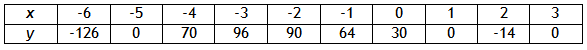Introduction

In certain situations, the table of values for a polynomial function is known, but the equation is not. To model a polynomial function using a table, work the steps in finding the x-intercepts of a polynomial backwards. First, determine the zeros of the function by looking at the table. Second, turn each zero into a factor of the function. Finally, determine the factored form of the polynomial.

Example 1 Determine the function that models the table below:Step 1. Determine the zeros of the function.

(-5, 0), (1, 0), (3, 0)

Step 2. Turn each zero into a factor of the function.

Since x = -5, then (x + 5) is a factor of the polynomial.

Since x = 1, then (x - 1) is a factor of the polynomial.

Since x = 3, then (x - 3) is a factor of the polynomial.

Step 3. Determine the factored form of the polynomial. Use a point from the table to find value of a.

y = a (x + 5)(x - 1)(x - 3); point (0, 30)
30 = a (0 + 5)(0 - 1)(0 - 3)
30 = a (15)
2 = a

y = 2 ( x + 5)( x - 1)( x - 3)